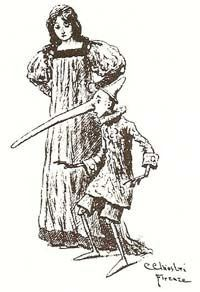# Card paradox

Updated on
Covid-19The card paradox is a non-self-referential variant of the liar paradox constructed by Philip Jourdain. It is also known as the postcard paradox, Jourdain paradox or Jourdain's paradox.

## The paradox

Suppose there is a card with statements printed on both sides:

Trying to assign a truth value to either of them leads to a paradox.

1. If the first statement is true, then so is the second. But if the second statement is true, then the first statement is false. It follows that if the first statement is true, then the first statement is false.
2. If the first statement is false, then the second is false, too. But if the second statement is false, then the first statement is true. It follows that if the first statement is false, then the first statement is true.

The same mechanism applies to the second statement. Neither of the sentences employs self-reference, instead this is a case of circular reference. See Yablo's paradox for a variation of the liar paradox that does not even rely on circular reference.

## References

Similar Topics
The Crazies (1973 film)
Brenda Wang
George Shirley
Topics

 B i Link H2
 L